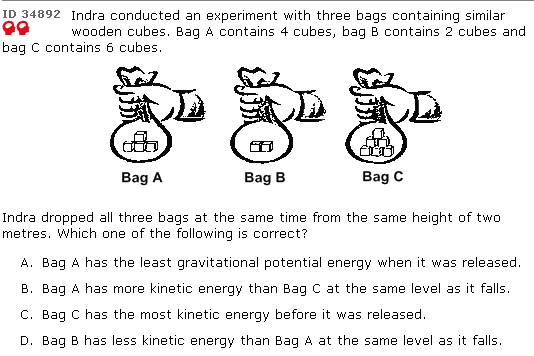# Science question – gravitational potential energyScience tip:

Gravitational Potential Energy – the more heavy the object and the higher it is above the ground, the larger its gravitational potential energy.

Kinetic Energy – the faster the object is moving and the more heavy it is, the greater its kinetic energy.

In this particular question – bag C starts off with the largest gravitational potential energy. followed by A and B.

The gravitational potential energy will be converted to kinetic energy when the bags are released.

(A) is false since B has the least.
(B) is false since C will have larger kinetic energy.
(C) is false because before it was released it wasn’t moving and hence kinetic energy is 0.
(D) is true.

(question source: oldschool)